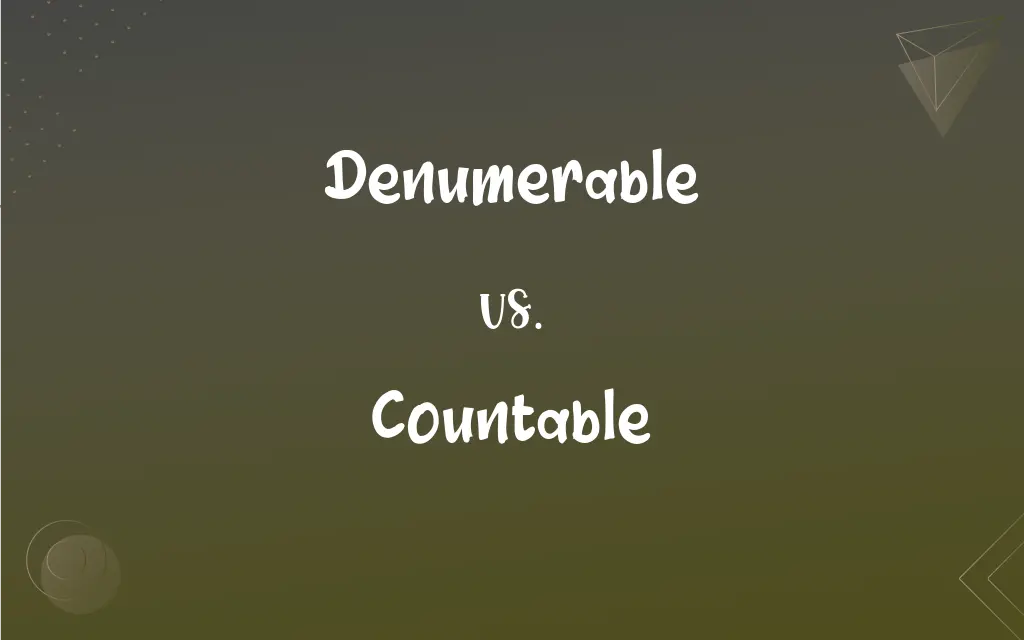# Denumerable vs. Countable: What's the Difference?## Denumerable and Countable Definitions

#### Denumerable

Capable of being put into one-to-one correspondence with the positive integers; countable.

#### Countable

Capable of being counted
Countable items.
Countable sins.

#### Denumerable

(mathematics) Capable of being assigned a bijection to the natural numbers. Applied to sets which are not finite, but have a one-to-one mapping to the natural numbers.
The empty set is not denumerable because it is finite; the rational numbers are, surprisingly, denumerable because every possible fraction can be assigned a natural number and vice versa.

#### Countable

(Mathematics) Capable of being put into a one-to-one correspondence with the positive integers.

#### Denumerable

That can be counted.

#### Countable

Capable of being counted; having a quantity.

#### Denumerable

That can be counted;
Countable sins
Numerable assets

#### Countable

Finite or countably infinite; having a one-to-one correspondence (bijection) with a subset of the natural numbers.

#### Countable

Countably infinite; having a bijection with the natural numbers.

#### Countable

Freely usable with the indefinite article and with numbers, and therefore having a plural form.

#### Countable

(grammar) A countable noun.

#### Countable

Capable of being numbered.

#### Countable

That can be counted;
Countable sins
Numerable assets